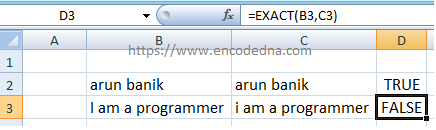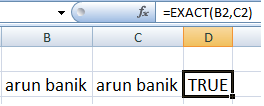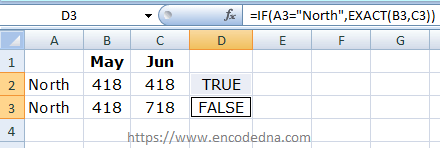﻿ Compare two Text Strings in Excel using EXACT Function

# Compare two Text Strings in Excel using EXACT Function

In Excel, you can use the EXACT() function to compare two text strings, to check if the texts are similar or not.## Syntax

EXACT(text1, text2)

The EXACT() function takes two parameters. See the syntax above. The parameters are two string values (if you are comparing numeric values, it will convert the values to text and compare).

Both parameters are required.

## Return Type

The function returns a Boolean True or False. So, if the comparing texts are similar, then the function will return TRUE or else it will return a FALSE.

Remember: The EXACT() function is case sensitive. Therefore, this is wrong -> Exact(). And, when comparing, it looks for texts taking into account the case.

## EXACT function examples

1) Comparing two strings

=EXACT("arun", "arun")     // This returns TRUE.
=EXACT("arun", "Arun")     // This returns FALSE. Its case sensitive, remember.

Now, in the above example, I have assigned values within double quotes. You can provide values explicitly to the function as parameters.

2) Comparing two numeric values

=EXACT("148", "1478")     // This returns FALSE.
=EXACT("148", "148")     // This returns TRUE.

Although, I am comparing numeric values, it will be converted to string values.

3) Comparing strings in worksheet cells

Now, I’ll compare texts (two strings) in my Excel worksheet. Let us assume the texts are in cells B2 and C2. The formula to compare will be,

=EXACT(B2, C2)4) Using EXACT function within IF statement

You can use the EXACT function within an IF condition or statement.

=IF(A2="North",EXACT(B2,C2))     //If value in A2 in North, then compare values in B2 and C2